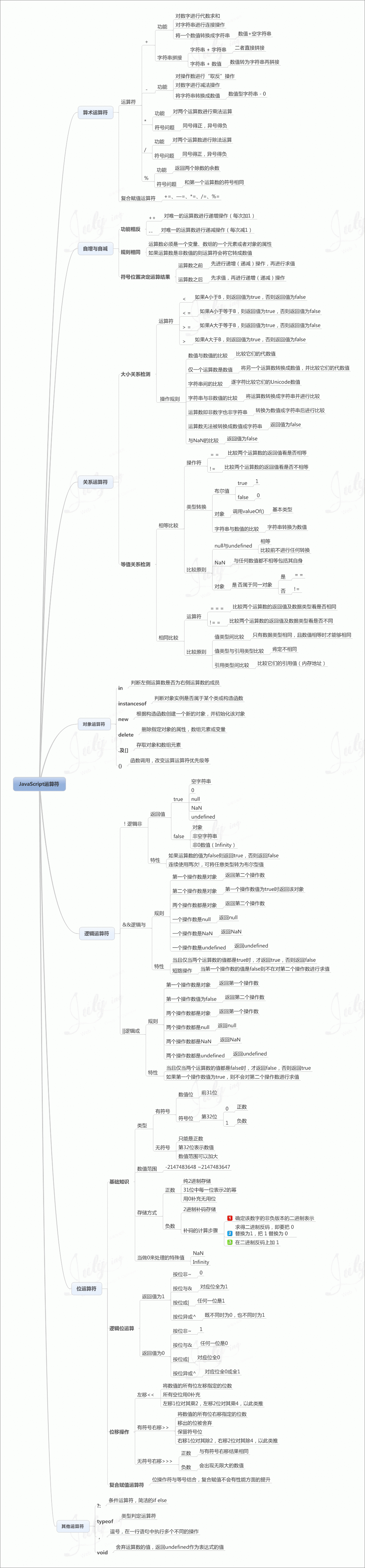# JavaScript 运算符

2021-11-06 14:49 更新

y=5;
z=2;
x=y+z;

7

## JavaScript 算术运算符

y=5

，下面的表格解释了这些算术运算符：

+ 加法 x=y+2 7 5 实例 »
- 减法 x=y-2 3 5 实例 »
* 乘法 x=y*2 10 5 实例 »
/ 除法 x=y/2 2.5 5 实例 »
% 取模（余数） x=y%2 1 5 实例 »
++ 自增 x=++y 6 6 实例 »
x=y++ 5 6 实例 »
-- 自减 x=--y 4 4 实例 »
x=y-- 5 4 实例 »

## JavaScript 赋值运算符

= x=y   x=5 实例 »
+= x+=y x=x+y x=15 实例 »
-= x-=y x=x-y x=5 实例 »
*= x*=y x=x*y x=50 实例 »
/= x/=y x=x/y x=2 实例 »
%= x%=y x=x%y x=0 实例 »

## 用于字符串的 + 运算符

+ 运算符用于把文本值或字符串变量加起来（连接起来）。

## 实例

txt1="What a very";
txt2="nice day";
txt3=txt1+txt2;

txt3 运算结果如下:

What a verynice day

## 实例

txt1="What a very ";
txt2="nice day";
txt3=txt1+txt2;

What a very nice day

## 实例

txt1="What a very";
txt2="nice day";
txt3=txt1+" "+txt2;

What a very nice day

x=5+5;
y="5"+5;
z="Hello"+5;

x,y, 和 z 输出结果为:

10
55
Hello5

## JavaScript运算符学习脑图## 参考文章

App下载